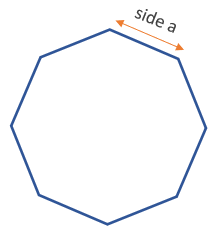# Area of an Octagon Calculator

Use this calculator to easily calculate the area of an octagon given the length of its side in any metric: mm, cm, meters, km, inches, feet, yards, miles...

Share calculator:

Embed this tool:
get code

## Area of an octagon formula

The formula for the area of an octagon of regular shape is 2 · (1 + √2) · side2, as seen in the figure below:The solution to the equation is straightforward and this is the formula used in our regular octagon area calculator. The result will be in whatever metric you did the measurement in, but squared: square mm, square cm, square dm, square meters or square inches, square feet, square yards, square miles etc. Note that this formula works for regular octagons only - if the octagon has an irregular shape, the calculation becomes significantly more complicated.

## How to calculate the area of an octagon?

The area of a regular octagon is fairly easy to calculate in that it requires just one measurement to be known - its side. One then replaces it in the equation above to find the area.

The relative simplicity of the octagon can be misleading when translated to real life where one needs to measure all sides and find them equal, before an octagon can be declared a regular one. Regular octagons can appear in construction, engineering, landscaping, etc.

## Example: find the area of an octagon

The only measurement needed to find the area of a regular octagon is its side. Since all sides are equal it does not matter which side is measured.

For example, if the side of a pool with the shape of an octagon is 10 yards, its area is then 2 · (1 + √2) · side2 = 4.828427 · 102 = 4.828427 · 100 = 482.8427 square yards.

#### Cite this calculator & page

If you'd like to cite this online calculator resource and information as provided on the page, you can use the following citation:
Georgiev G.Z., "Area of an Octagon Calculator", [online] Available at: https://www.gigacalculator.com/calculators/octagon-area-calculator.php URL [Accessed Date: 29 May, 2023].